# Calculus

 1 Introduction to Calculus 2 Functions

## Introduction

Calculus was originally called infinitesimal calculus or "the calculus of infinitesimals."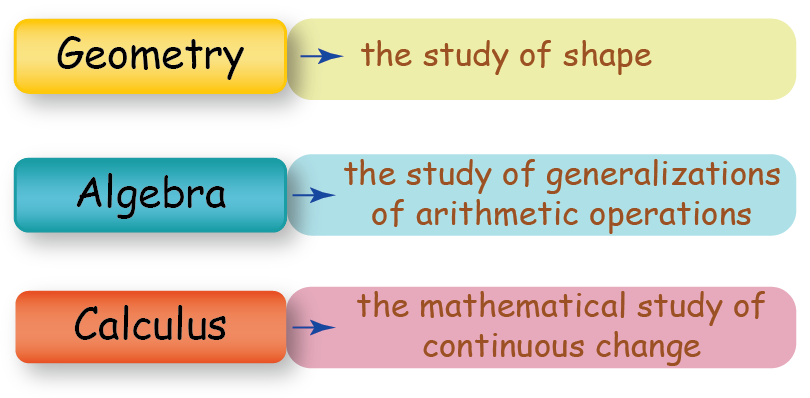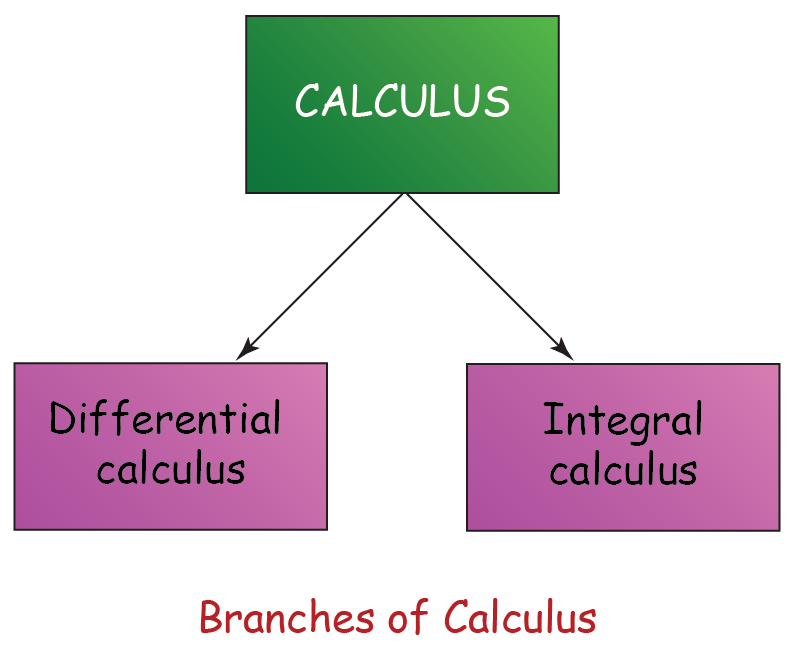You can go ahead and explore Functions in Calculus.

## Functions

Functions represent the relationship between two variables, which are the independent variable and the dependent variable.

Let’s consider the following diagram.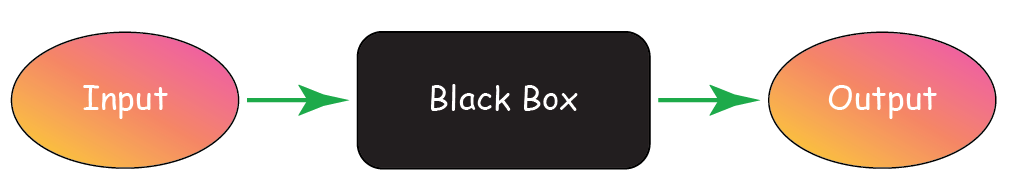There is an INPUT, a black box, and an OUTPUT.

Suppose we want to make a Pizza.

We would need the following basic ingredients.

• Pizza Base
• Pizza Sauce
• Cheese
• Seasoning

Using the diagram given above, we get: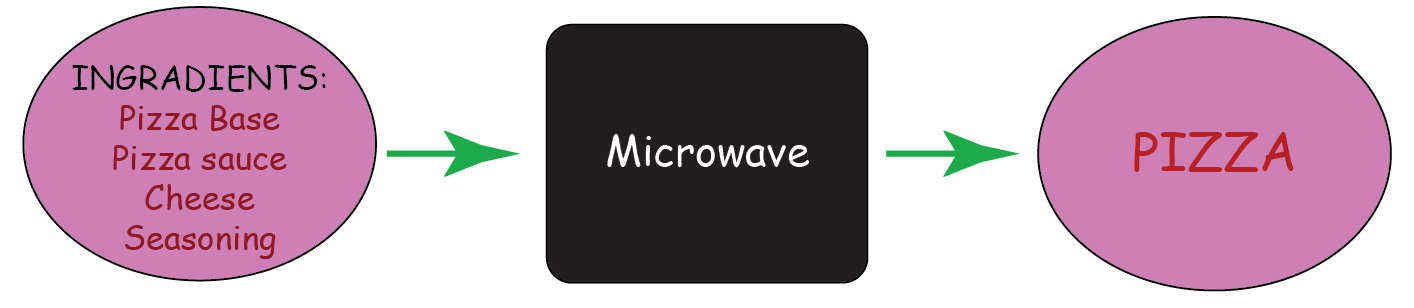The taste of our Pizza depends on the quality of the ingredients.

Let’s take another example

Suppose that: $$y = x^2$$

 Value of $$x$$ Value of $$y$$ $$1$$ $$1$$ $$2$$ $$4$$ $$9$$ $$81$$ $$11$$ $$121$$

Using the diagram given above, we get: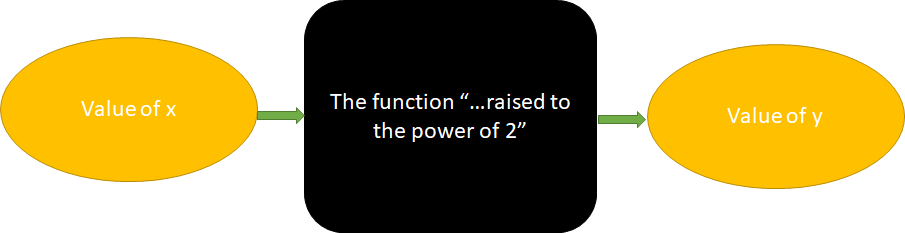We can see that the value of $$y$$ depends on the value of $$x.$$

We can conclude that

-  the INPUT is independent of the OUTPUT
-  the OUTPUT depends on the INPUT
-  the Black Box is responsible for the transformation of the INPUT to the OUTPUT

In calculus,

-  the INPUT is an independent variable
-  the OUTPUT is a dependent variable
-  the Black Box is a function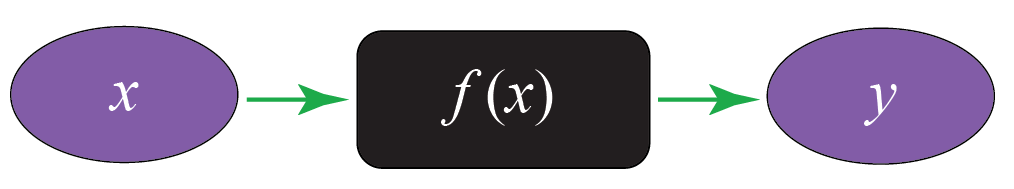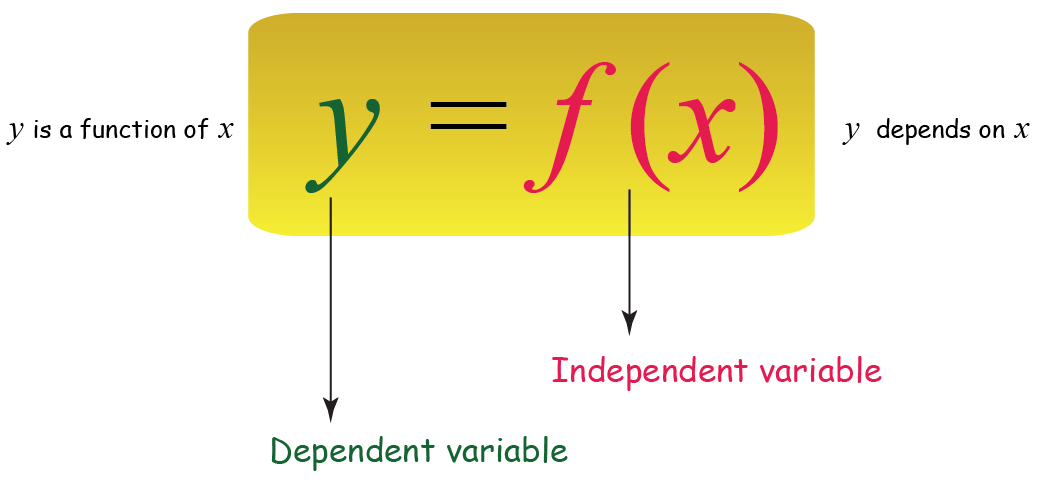Now let us study some other concepts of functions such as Introduction to the Concept of Functions, Visualizing Functions through Graphs, Domain and Range of a Function, Restrictions on Domain, Piecewise-Defined Functions, Constant Functions, Linear Functions, Quadratic Functions, Polynomial Functions, Exponential Functions, Modulus Function, Reciprocal Function, and the Greatest Integer and Fractional Part Functions.

We will also study in detail the concepts of Vertical Translation, Horizontal Translations, Vertical Scaling, Horizontal scaling, Applying modulus, and Graphing a Quadratic Function.